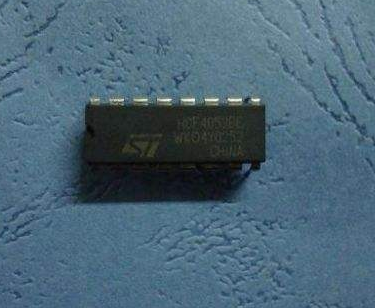# Maxim's new analog switches simplify data acquisition system calibration tasks

In the data acquisition system, the various components of the front-end analog channel—sensors, signal conditioning circuits, and analog-to-digital conversion systems—will bring errors to the measurement results to varying degrees, and the error will drift with temperature and time. The errors and drifts of sensors and signal conditioning circuits have received extensive attention and a variety of techniques have been developed to calibrate and compensate them. For example, the pressure sensor can be compensated for output accuracy of 0.1% or higher. But for the last part of the channel - the error caused by the analog to digital converter is often overlooked. The error of the linear system is divided into three types: zero (offset) error, gain (fullness) error, and nonlinear error. Analog-to-digital converter ADCs typically have excellent linearity, such as the popular 12-bit A/D converter AD574 with non-linearity errors (INL and DNL) within 1 LSB. However, the zero and gain errors are not ideal. Typical 12-bit A/D converters can have up to 10 LSB errors, which is unacceptable for data acquisition systems that require 0.1% accuracy. Of course, you can use the trimmer potentiometer method to correct these two errors, you can also consider the use of high-resolution converters. But the former increases the complexity of the line adjustment, which increases the cost of the product.Another solution is to calibrate the analog-to-digital converter in software using a calibration channel. But this requires at least two high-precision standard signals. It is difficult to obtain a standard voltage signal with an accuracy of more than 0.1% using a discrete solution, and the problem remains unresolved.

Maxim's analog switch with "calibration" ("calibration multiplexer") MAX4539 solves this problem. This novel analog switch utilizes laser trimming technology to integrate a high-precision resistor divider network with a voltage divider ratio accuracy of 0.002% (better than 15 bits), providing the ADC with the high required for zero calibration and gain calibration Precision standard signal. A low-precision voltage divider network is also integrated inside the chip to monitor system power. This makes it easy to implement system self-tests, data acquisition, and system calibration without adding any additional hardware.

MAX4539 structure and working mode

The internal structure of the MAX4539 is shown in Figure 1. The main part is a common low voltage, 8-channel CMOS analog switch. There is also a resistor divider network. The voltage divider network divides the externally input reference voltage or supply voltage and sends it to the multiplexer output through the selector switch. The decoding circuit decodes the control signal and the address signal and controls the internal switch to realize input channel selection or operation mode switching.

The MAX4539 has three modes of operation: self-test and self-test. Different operating modes can be selected via control pin CAL and address pins A0~A2. In addition, it has enable control and status latching. It is very simple to interface with the CPU. See the truth table for the selection of operating modes and input channels.

When CAL is inactive (low level), the device operates in measurement mode, which is equivalent to an ordinary low-voltage, 8-to-1 analog switch. The input channel is selected by address A0~A2. When CAL is active (high level), the chip enters self-test and self-calibration mode, and different calibration or self-test functions can be selected through A0~A2. A2A1A0=101 selects the gain calibration mode, the output voltage is VCOM=4081/4096(VREFHI-VREFLO)±0.0024%, where VREFHI and VREFLO are the voltages of the input REFHI and REFLO pins, which are generally used by analog-to-digital converters. The reference voltage. For ideal A/D, the result of this voltage conversion should be 4081. According to the deviation between the actual conversion result and this value, the gain error of A/D can be calculated. When A2A1A0=110, the zero point calibration is performed, and the output is VCOM=15/4096. (VREFHI-VREFLO) ± 0.0024%, calculate the zero error of A/D according to the deviation between the actual conversion result and 15; perform system self-test when A2A1A0=000 or 011, the internal voltage network samples the power supply voltage and sends it to A/D. For the detection, the sample values ​​are: VCOM = 1/2 (V +) ± 0.4% or 5 / 8 (V + - V -) ± 0.4%. Alternatively, the voltage at REFHI, REFLO or GND can be sent to the A/D for detection.